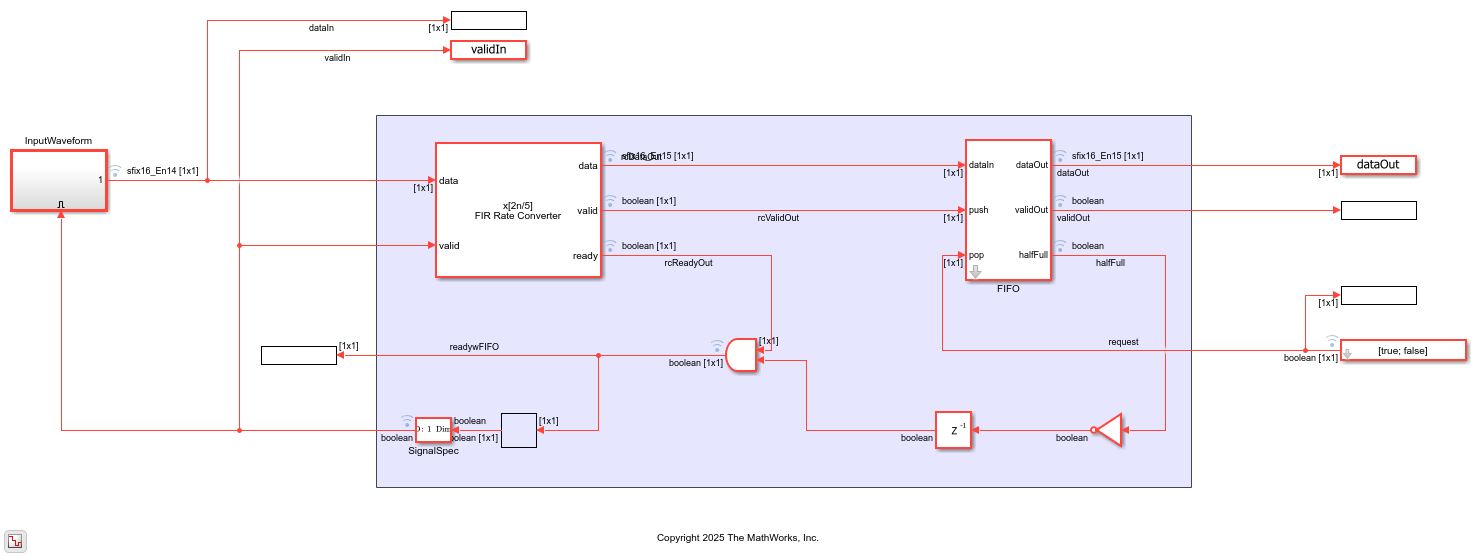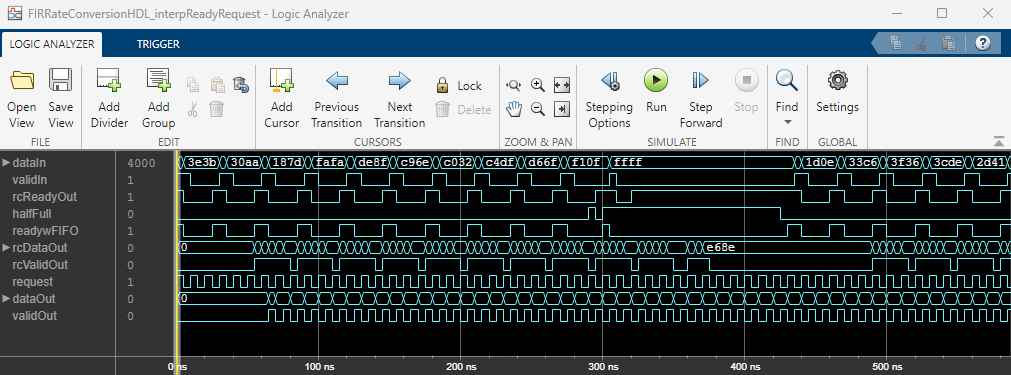# Control Data Rate Using Ready Signal

This example shows how to use a backpressure signal to control an upstream data source for an interpolator design.

It also shows how to regulate the output data pattern by using a FIFO and a request signal.

Hardware algorithms operate on streaming data to make efficient use of hardware resources, such as memory for storing samples. The blocks have limited storage for incoming samples, and take time to process each sample. In an interpolator design, there must also be cycles available to accommodate the interpolated output samples.

To ensure that a block does not receive input samples when it cannot accept them, use one of these options.

• Space the input samples with invalid cycles in between to accommodate the algorithm. Regular input spacing can also enable internal resource sharing. For details of this type of input rate management, see the Implement Digital Upconverter for FPGA example.

• Use a backpressure signal to stop upstream blocks from providing data when downstream blocks cannot accept it. DSP HDL Toolbox™ blocks provide the `ready` output signal to indicate when the algorithm can and cannot accept new input samples. This example shows how to use this signal to implement backpressure in your system.

The example model uses a FIR Rate Converter block to upsample a signal from 40 MHz to 100 MHz. To achieve this rate change, the block must return 2 or 3 output samples for each input sample. The generation of these extra samples means that the block cannot accept new input samples every cycle, and that the output rate can be nonuniform. This example shows how to use the `ready` output signal to control the upstream data source, and how to use a FIFO to regulate the output data rate.

### Configure Model

Define the data rate parameters. The Simulink® model uses these values to configure the FIR Rate Converter block and the FIFO control signals. Use the `firpm` function to design an equiripple FIR filter.

```FsIn = 40e6; % input signal sample rate TsIn = 1/FsIn; % input signal sample time NsIn = 100; % number of input samples t = TsIn*(0:NsIn-1); % input time instants stopTime = (NsIn-1) * TsIn; Ts = 1/200e6; % system clock rate; twice the output sample rate coeffs = firpm(70, [0,.15,.25,1], [1,1,0,0]); interpFactorL = 5; decimFactorM = 2; ```

The output FIFO depth must be at least two times the delay around the feedback loop in cycles. This delay is equal to the number of cycles between the `request` signal going to 1 (`true`) to pop the FIFO (enabling a new input to the FIR Rate Converter block) and the resulting output `valid` from the FIR Rate Converter block.

The latency of the block is a pipelined adder tree for the filter coefficients, plus 7 cycles. When interpolating, each input sample can yield up to `ceil(L/M)` output samples. The feedback loop also includes one cycle for the FIFO to update, one cycle for `ready` computation, and one cycle for the register on the feedback path.

```delayLineLength = ceil(length(coeffs)/interpFactorL); rateConverterLatency = ceil(log2(delayLineLength)) + 7; feedbackLoopLatency = rateConverterLatency + ceil(interpFactorL/decimFactorM) + 3; fifoDepth = 2*feedbackLoopLatency; ```

In the model, the FIR Rate Converter block and the FIFO subsystem are configured by using the workspace variables already defined. The FIR Rate Converter block has the optional ready port enabled and the coefficient data type is set to `fixdt(1,16,15)`.

```modelName = 'FIRRateConversionHDL_interpReadyRequest'; open_system(modelName); set_param(modelName,'SimulationCommand','Update'); ```To control the input data rate, the block sets the `ready` output signal to 1 (`true`) when the block can accept a new input sample on the next time step. Upstream blocks must only apply valid input data when the `ready` signal is 1 (`true`). This example model combines the `ready` signal with a signal that indicates when the output FIFO is less than half full. The model connects the combined signal as an enable to a waveform source that generates one input sample at a time. This input data rate control ensures that data is only applied when the block is ready to accept it, and that the output FIFO will not overflow.

To control the output data rate, the model implements a FIFO for the output samples. The model connects a toggling signal to the FIFO pop port. When you use a system clock of 200MHz and return output samples from the FIFO every second time step, the output data rate is a uniform 100 MHz.

### Run Model and Display Results

Open the Logic Analyzer to view the input and output signals of the block. The blue icon on signals in the model indicates those signals are logged and available to view in the Logic Analyzer.

The waveform shows how the `ready_before_and` signal toggles when the block is processing input samples and cannot accept new data. The FIFO `halffull` signal also turns off the combined `ready` signal to the input generator. The `validIn` signal responds to the combined `ready` signal to avoid sending new data when either the block or the FIFO does not have room. The waveform shows that the `request` signal is high every second cycle, which generates the final `dataOut` and `validOut` signals from the FIFO.### Generate HDL Code

You must have the HDL Coder™ product to generate HDL code. To generate HDL code from the FIR Rate Converter block and the FIFO and ready logic, create a subsystem from the area shown in the model. Then right-click the subsystem and select HDL Code > Generate HDL Code for Subsystem.